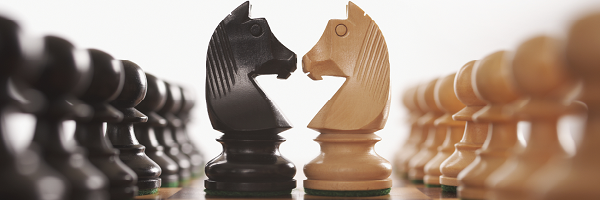# Playing with PowersCharlie chose some pairs of numbers, squared them, and added them together:

$3^2 + 3^2 = 18$

$15^2 + 6^2 = 261$

$9^2 + 21^2 = 522$

His answers were all multiples of $3$.

Can you find some other pairs of numbers whose squares add up to a multiple of 3?

Can you explain why?

Alison has also been choosing pairs of numbers, squaring them, and then adding them together.

Her answers were all multiples of 4.

Can you find some pairs of numbers whose squares add up to a multiple of 4?

What is special about these pairs of numbers?

Can you explain why?

Can you find pairs of square numbers that add up to multiples of 5?

Julia wonders if $3^{444}+4^{333}$ is a multiple of $5$.

Her calculator can't help her out.

Can you?